# TRIGONOMETRY: SINE RULE AND COSINE RULE

Introduction

The solution for an oblique triangle can be done with the application of the Law of Sine and Law of Cosine, simply called the Sine and Cosine Rules. An oblique triangle, as we all know, is a triangle with no right angle. It is a triangle whose angles are all acute or a triangle with one obtuse angle.

The two general forms of an oblique triangle are as shown:

 Sine rule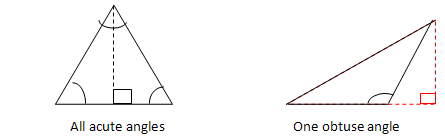## Sine Rule (The Law of Sine)

The Sine Rule is used in the following cases:

CASE 1: Given two angles and one side (AAS or ASA)

CASE 2: Given two sides and a non-included angle (SSA)

The Sine Rule states that the sides of a triangle are proportional to the sines of the opposite angles. In symbols,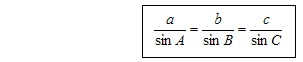## Case 2: SSA or The Ambiguous Case

In this case, there may be two triangles, one triangle, or no triangle with the given properties. For this reason, it is sometimes called the ambiguous case. Thus, we need to examine the possibility of no solution, one or two solutions.

## Cosine Rule (The Law of Cosine)

The Cosine Rule is used in the following cases:

1. Given two sides and an included angle (SAS)

2. Given three sides (SSS)

The Cosine Rule states that the square of the length of any side of a triangle equals the sum of the squares of the length of the other sides minus twice their product multiplied by the cosine of their included angle. In symbols: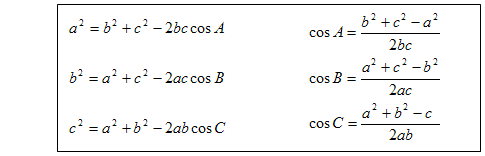NB: after this lesson you will be able to apply the sine rule for triangles to calculate an unknown side and unknown angle of a given triangle.

Use the link below for more details on when to use sine and cosine rule

Click the links below and watch the videos to assist you in completing this task in sine an cosine rule.

https://www.savemyexams.co.uk/notes/cie-igcse-maths-new/6-trigonometry-cie/6-4-sine-cosine-rule-cie/6-4-1-sine-cosine-rules-area-of-triangle-basics-cie/

Questions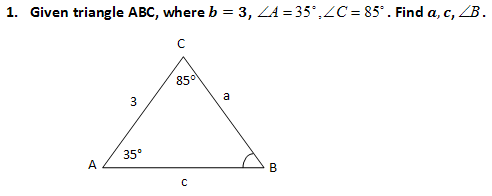2.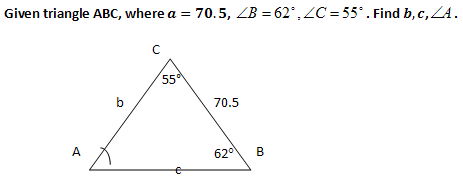3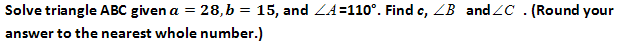4.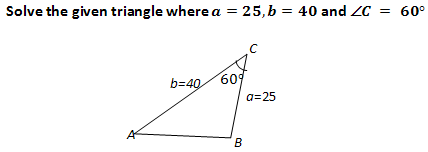5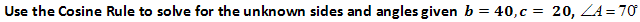6. Prove the Sine rule and the cosine rule

Process
1. Read the introduction to see formulas for sine and cosine rule.
2. Watch all the videos to be able to answer the above questions
4. Watch this video to answer some of the questions

Evaluation
 Questions Angle mark Side mark Total 1 3 4 7 2 3 4 7 3 2 8 10 4 2 8 10 5 2 8 10 Total = 44

6. 4 marks for each rule proven

Total mark : 52 Marks

Conclusion

In this lesson we have proven the sine and the cosine rule.

We applied the laws in finding the unknown angles and the unknown sides.

We can identify situation where the laws can used.

We will continue with the area rule on our next session.

watch the video below for the next session

Credits
Teacher Page

To solve a triangle is to find the lengths of each of its sides and all its angles. The sine rule is used when we are given either a) two angles and one side, or b) two sides and a non-included angle. The cosine rule is used when we are given either a) three sides or b) two sides and the included angle.

Lusanda Mpopoma-Sophazi

209075031